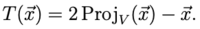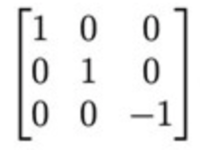# Linear algebra, determine a matrix representation A for the image of T.

#### Karl Karlsson

##### New member
The plane V in R3 is given by the equation 2x + 3y + 5z = 0. The transformation
T: R3 -> R3 is given by:Determine the matrix representation A for the image of T.